# thesIt

##Updates from ArifRSS

• #### 06:32:12 am on August 22, 2010 | 0 | # | Tags: basic, density, wikipedia

Density of a material is defined as its mass per unit volume.
ρ (in some countries i.e. USA) is also defined as weight per unit volume
Units: (kg/m³), g/cm³

• #### 01:42:26 am on August 22, 2010 | 0 | # | Tags: book, Soong 2004, standard citation

Soong TT. 2004. Fundamentals of Probability and Statistics For Engineers. West Sussex: J Wiley.

• #### 06:29:08 pm on August 21, 2010 | 0 | # | Tags: cross-validation, Fu 1994, k-fold, validation

../sT-fold cross-validation (Stone 1974) repeats k times for a sample set randomly divided into k disjoint subsets, each time leaving one set out for testing and the others for training. Thus, we may call this technique “leave some out”

• #### 07:50:38 pm on August 11, 2010 | 0 | # | Tags: Enăchescu 2008, standard citation

Enăchescu C. 2008. Approximation capabilities of neural networks. JNAIAM 3(3-4):221-230.

• #### 07:27:51 pm on August 11, 2010 | 0 | # | Tags: Enăchescu 2008, neural network, universal approximation property

Approximation Capabilities of Neural Networks. C. Enăchescu, Journal of Numerical Analysis, Industrial and Applied Mathematics (JNAIAM), vol. 3, no. 3-4, 2008, pp. 221-230

From the learning point of view, the approximation of a function is equivalent with the learning problem of a neural network. In this paper we want to show the capabilities of a neural network to approximate arbitrary continuous functions. We have made some experiments in order to confirm the theoretical results.

c48613aed54c29e0f60ca35833aebf70.pdf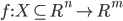$f : X \subseteq R^{n} \to R^{m}$ is a continuous function

## Neural Network and Best Approximation Theory

Given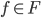$f \in F$ and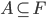$A \subseteq F$ we call the distance of f from A as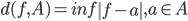$d(f,A)=inf\left \| f-a \right \|, a\in A$

• #### 10:27:38 pm on June 28, 2010 | 0 | # | Tags: basic, standard deviation, statistics, variance

Easiest description for standard deviation definition is distance from mean (expected value) as shown in this graphical depictionwhere all the values fall at σ distance within the dotted circle radius. Of course a more real-life situation is shown aswhere σ is the square root of the following mean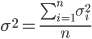$\sigma^2 =\frac{\sum_{i=1}^{n}{\sigma_{i}^2}}{n}$

σ² a.k.a. variance is averaged quadratic distances. Explanation:

Distance may have several concepts, in this variance description, distance shows “how far” a value is from its population expected value (mean). Quadratic form of this “how far” is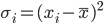$\sigma_i=(x_i-\bar{x} )^2$

• #### 12:02:50 pm on April 18, 2010 | 0 | # | Tags: analysis, data processing

Between different sulfonation which results in different IFT, standard deviation of these parameters are
viscosity : 21.0 - 24.7 pH : 0.11 - 0.13 density : 0.015 - 0.018 IFT : 0.015 - 0.033 

### (historical effort)

A plot showing surface tension as function of pH showed quite similar relative changes in pH 5-9. Johlin measured using sessile bubble method with different timing (hours) to expect equilibrium.

However, the pattern isn’t the same as Sugden’ result that was imposed over the same plot.

• #### 03:39:24 pm on February 27, 2010 | 0 | # | Tags: abstract, Danielli 1937, interfacial tension, pH, reference

Danielli JF. 1937. The Relations between Surface pH, Ion Concentrations and Interfacial Tension. Proceedings of the Royal Society of London. Series B, Biological Sciences, Vol. 122, No. 827 (Apr. 1, 1937), pp. 155-174

abstract here

• #### 03:31:28 pm on February 27, 2010 | 0 | # | Tags: abstract, interfacial tension, Petelska 2002, pH, reference

Petelska AD, Naumowicz M, Figaszewski ZA. 2002. Interfacial tension of the bilayer lipid membrane. Cell Moll Biol Lett 7:212.

A regularity has been found: a larger hydrophilic head gives rise to a lower interfacial tension. A relationship was found between the size of the hydrophilic head of lipid and the isoelectric point pH value. With larger hydrophilic heads, the isoelectric point appears at lower pH.

abstract:
Vol7_suppl_05_39.pdf

« Previous PageNext Page »